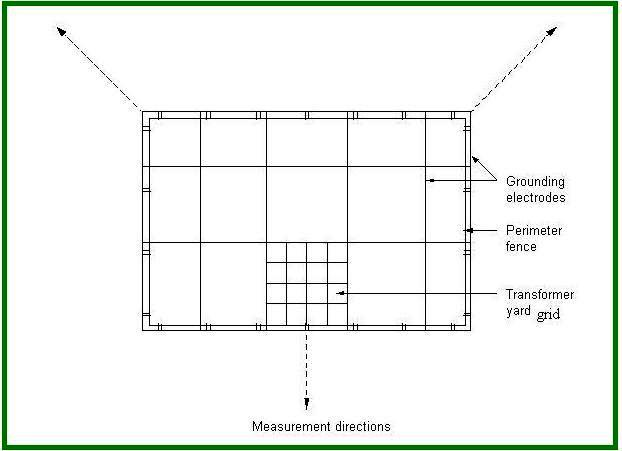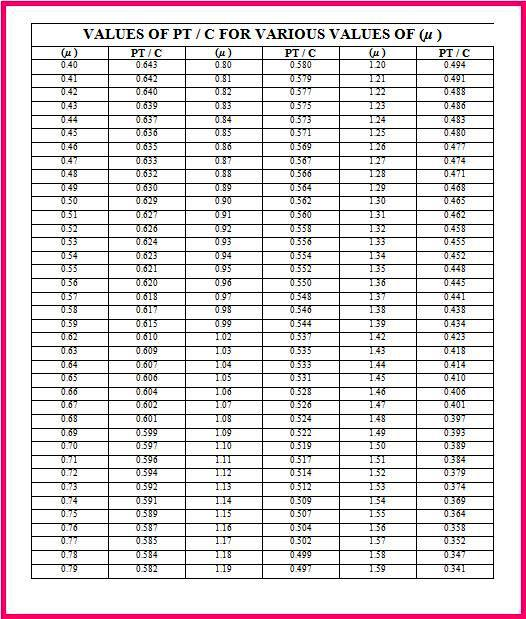## Pages

### 1 How to Calculate Ground Resistance

In Article " Identifying Grounding Connectors and Bushings ", I explained the two methods for making the grounding connections, which were:

• Soldered Splice,
• Solderless Splice (Compression Connectors).

Today, I will explain Practice#1: How to Determine Ground Resistance as follows.

You can review the following Articles For more information:

 Practice#1: How To Determine Ground Resistance

 Practice#1A: How To Measure Ground Resistance

To Measure Ground Resistance, Follow the steps in this Practice to measure ground resistance for a grounded system using a megohmmeter.

1- Locate perimeter electrodes of the grounded system you will use as test electrodes. There are three locations as shown in Figure 1.Figure 1. Test electrode measurement directions

2- Determine probe (CP) distance location from grounded system.

Example#1:

If the substation is 150 X 200 meters, Determine probe (CP) distance location from grounded system.

Solution:

So, The distance of CP should be 250 meters from the electrode(s) tested.

Note: Where no direct ground system size measurements can be determined or for a rough estimate, use the minimum 100 m spacing.

3- Verify that the correct equipment is used for measuring

4- Set up equipment as shown in Figure 2, driving probes to a depth of 2 feet (0.6 m) minimum, into the ground.Figure 2. Meter setup for fall-of-potential measurement

Warning: Severe shock can occur if equipment safety procedures are not followed correctly.

5- Resistance readings at potential probe spacing equal to 0.2 C, 0.4 C, and 0.6 C, are taken as shown in Figure 3.These are resistances R1, R2, and R3 respectively.Figure 3. Probe location with resistance graph comparison

6- Calculate the slope variation coefficient using the following formula:

7- Interpret the results.Figure 4. Table of values for PT / C related to µ

With µ calculated, and found to be   ≤ 1.59, the value of PT/C is taken from the table of values in Figure 4. If µ is not within this range, extend the current probe farther from the grid.
PT will be the distance of the potential probe position to the test grid where the true resistance should be measured.
Measure the true resistance by inserting the potential probe at the corresponding distance PT or µ (c).

Example#2:

Determine the CP probe distance from a grounded system that is 300m X 200m in area.

Solution:

Using the formula,Example#3:

Repeat the same in example#2 but with a grid measuring 150m X 175m.

Solution:Example#4:

Resistance readings at potential probe spacing equal to 0.2 C, 0.4 C, and 0.6 C, are taken. These correspond to resistances R1, (0.4) R2, (0.8) and R3 (1.2). What is the resistance measurement and where should the PT probe be placed to obtain the true value?

Solution:Example#5:

Resistance readings at potential probe spacings were found to be; (0.6) (0.85) (1.12), what is the location of Pt.

Solution:Example#6:

Calculate µ for the location and determine where the true value should be measured. Values given are (0.5) (1.1) and (2.05).

Solution:

 Practice#1B: How to Estimate Minimum Ground Grid Resistance

 Follow the steps in this Practice to calculate the Minimum grid resistance as follows: Calculate the Minimum ground resistance of the grid using the following formula: Where: Rg  = ground resistance in Ω   ρ  = average earth resistivity in Ω/m   A  = area occupied by the ground grid in m2   π  = 3.14

Example#7:

Calculate the Minimum ground resistance of a Transformer Yard grid 100 x 70 meter, knowing that ρ = 750 Ω/m.

Solution:

Using the formula:

Example#8:

Calculate the Minimum ground resistance (Rg) of the grid if soil resistivity is 2000 Ω/m for a ground grid 100 x 60 meter.

Solution:

A = 100 m x 60 m = 6000 square meters

Using the following formula:

 Practice#1C: How to Estimate Upper Limit of Ground Resistance

 Follow the steps in this Practice to calculate Upper Limit of Ground Resistance as follows: Calculate the Upper Limit of Ground Resistance of the grid using the following formula: Where: Rg = station ground resistance in Ω Ρ = average earth (soil) resistivity in Ω/m A = the area occupied by the ground grid in m2 L = the total buried length of conductors in m Π = 3.14

Example#9:

Calculate the Upper Limit of ground resistance (Rg) of the grid if soil resistivity is 750 Ω/m for a ground grid shown in below figure.

Solution:

A = 11250 m2

L = 1200 m

To solve for Rg use the formula and plug in the above figures.Example#10:

Calculate the Upper Limit of ground resistance (Rg) of the grid if soil resistivity is 180 Ω/m for a ground grid shown in below figure.Solution:

L (Total length grounding grid conductors) = (4 x 200) + (6 x 150) + (6 x 50) = 2000 meters

A = 200 x 150 = 30,000

Plugging in the numbers for the formula:In the next article, I will explain Other Practices for Inspection of ground Grids, Rods, and Fence grounds. Please, keep following.

#### 1 comment:

1.(4 x 200) + (6 x 150) + (6 x 50) plz explain this why 4 and 6 multiplier?